# Algebra 1 : How to find mode

## Example Questions

← Previous 1 3 4 5 6 7 8 9 16 17

### Example Question #1 : How To Find Mode

When rolling two standard six-sided dice, what is the probability of NOT getting a seven?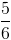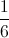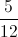Explanation:

When rolling two six-sided dice there arepossible outcomes.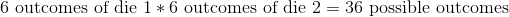The possible combinations to get a seven are: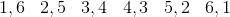.

So there are six ways to get a seven, out ofcombinations total. The resulting probability of getting a seven is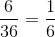.

The probability of NOT getting a seven is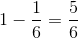.

### Example Question #2 : How To Find Mode

A short quiz with 5 questions is given to a class of 30 students. 7 students answered 5 questions correctly, 12 students answered 4 questions correctly, 9 students answered 3 questions correctly, and 2 students answered 2 questions correctly.

What is the mode among the class's scores?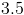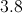Explanation:

The mode is the value that appears most often in a set of data. Within the class, 7 students answered 5 questions correctly, 12 students answered 4 questions correctly, 9 students answered 3 questions correctly, and 2 students answered 2 questions correctly. More students scored a 4 than any other score, so this score is the class's mode.

### Example Question #3 : How To Find Mode

Which of the following data sets has exactly one mode regardless of the value of?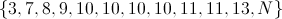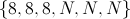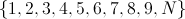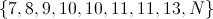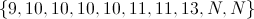Explanation: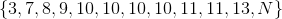is the correct set; no matter whatis, no value other than 10 can appear four or more times.

To see that no other choice works, let's examine them.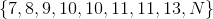: Ifis any number other than 10 or 11, then the number has 10 and 11, and possibly one other number, as modes, each appearing twice.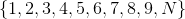: Ifis not any of the numbers already in the set, the set has no repeated values and thus no mode.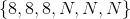: If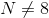, then the set is bimodal, with 8 andthe modes.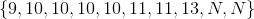: If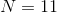, the set is bimodal, with 10 and 11 the modes.

### Example Question #4 : How To Find Mode

Find the mode of the following set of numbers: 2, 8, 4, 9, 2.Explanation:

The mode of a set of numbers is the number that appears the most.  In this set of numbers, 2 appears twice, and all of the other numbers appear only once.  Thus, 2 is the mode of this set of numbers.

### Example Question #5 : How To Find Mode

A teacher is grading quizzes and recording the scores in her records.  The scores are recorded as follows: 81, 91, 83, 88, 74, 98, 81, 94, 68, 92, 77, 79, 83, 91, 81, 84, 85, 81, 85, 79, 83, 81, 83.

What is the mode?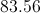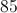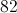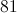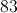Explanation:

The mode of a set of statistics is simply the most commonly occuring observation. In this case, what was the most common score on the quiz? We have 5 instances of 81, which is the most occurences of any particular number, thus our mode is 81.

### Example Question #6 : How To Find Mode

Determine the mode of the set.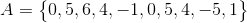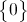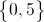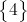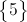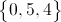Explanation:Mode refers to the number occuring most often, so in this example we are looking for the numbers that repeat and how many times they repeat.repeats twicerepeats twicerepeats twice

Now because all three numbers repeated an equal amount of time, all three then become our mode.

Answer :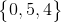### Example Question #7 : How To Find Mode

We have the following set of numbers:

15, 14, 17, 17, 12, 19, 14, 10, 12, 17, 18, 9, 16, 12, 17.

Find the mode.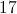Explanation:

The mode is the number that appears the most in the number set. To figure out what number appears the most in our number set, it would help to rearrange the numbers so that the set is rewritten as

9, 10, 12, 12, 12, 14, 14, 15, 16, 17, 17, 17, 17, 18, 19

By looking at this number set, it seems that the number 17 is the most common number in the number set.  It appears 4 times while other numbers such as 12 appear three times.  Thus, 17 is the mode.

### Example Question #8 : How To Find Mode

If you grab a pen at random from a box containing 4 red pens, 3 blue pens, and 5 black pens, what is the probability of choosing a blue pen?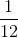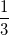Explanation:

There are 12 total pens (4 + 3 + 5 = 12). There 3 blue pens of the 12 total. The chances of grabbing a blue pen are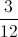, which reduces to.

### Example Question #1 : How To Find Mode

What is the mode of the following data set?

{9, 10, 4, 11, 7, 5, 15, 7, 2, 20, 11, 19, 7, 9}

11

7 and 9

2

9

7

7

Explanation:

Arrange the set in numerical order: {2, 4, 5, 7, 7, 7, 9, 9, 10, 11, 11, 15, 19, 20}.

You can then chart the frequency of each value.

Value (Frequency)

2 (1)

4 (1)

5 (1)

7 (3)

9 (2)

10 (1)

11 (2)

15 (1)

19 (1)

20 (1)

From this you can see the most common value, which is the mode, is 7.

### Example Question #2 : How To Find Mode

A set comprises ten elements, which are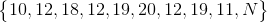wherestands for an unknown number.

What canbe set to in order to make the set bimodal?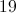The set cannot be bimodal for any value of N.Explanation:

12 appears in the set most frequently, appearing three times; 19 is second in frequency, appearing twice. 10, 11, 18, and 20 appear once each.

The only number we can setto to make two data values tie for appearing most frequently is 19; 12 and 19 would then each appear three times, and the others, once. Ifis set to any other number, 12 would remain the most frequent data value.

← Previous 1 3 4 5 6 7 8 9 16 17

### All Algebra 1 Resources